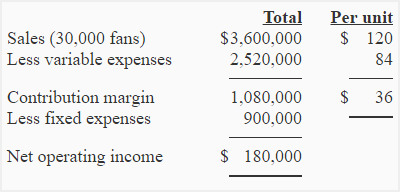# Exercise-4 (Degree of operating leverage, contribution margin income statement)

TLK Ltd. manufactures small size fans to be used in load shedding areas. Each fan has a rechargeable battery and a built in charging circuit. TLK sells a fan for \$120. The annual sale is 30,000 fans. Variable and fixed cost data is given below:

• Variable expenses: \$84 per fan
• Fixed expenses: \$900,000 per year

Required:

1. Prepare contribution margin income statement and compute the degree of operating leverage.
2. Next year the sales are expected to increase by 7,500 fans. Compute (a) the expected percentage increase in net operating income (b) expected increase in net operating income and (c) expected total net operating income for the next year. (You don’t need to prepare an income statement, use the degree of operating leverage for your answers).

## Solution:

### (1) Income statement:Degree of operating leverage = Contribution margin / Net operating income

= 1,080,000 / 180,000

= 6

### (2) Expected increase in net operating income:

The degree of operating leverage (computed above) is 6. The net operating income is expected to increase 6 times as fast as the sales of TLK company.

#### (a). Expected percentage increase in net operating income:

(Percentage change in sales × 6)

= *25% × 6

= 150%

*\$7,500/\$30,000 = 0.25 or 25%

#### (b). Expected increase in total net operating income:

Since the degree of operating leverage is 6, a 25% increase in sales will translate into 150% increase in net operating income.

= \$180,000 × 1.50

= \$270,000

#### (c). Expected total net operating income:

= \$180,000 + \$270,000

= \$450,000

A D V E R T I S E M E N T
3 Comments on Exercise-4 (Degree of operating leverage, contribution margin income statement)
1.Chris

Thank you very much, i had no idea where or how or the relevance of Degree of Operating Leverage. I’m ready for this test!

2.Abdullah Al Masud

Very helpful me.but exercise more for effective learning for students.

3.Sulaiman Freeman

my best web site